# Making samples linearly separable with an SVM¶

#### Matt Luther¶

In this post, we'll see a standard basic example of a data set which is not linearly separable. We'll see how computing extra features from the given data can allow that data set to be linearly separated.

First, we'll import what we need.

In :
%matplotlib inline
import numpy as np
import matplotlib.pyplot as plt
from mpl_toolkits.mplot3d import Axes3D
from sklearn.svm import LinearSVC


## The example data¶

We'll construct a set with two classes of points. The goal is to construct a set with one class of points in a central cluster, and another class of points in a ring around that cluster.

In :
n_samples = 200
# the feature matrix and label vector that we will populate later
features = np.zeros((n_samples,2))
labels = np.zeros((n_samples,1))
# info for the normal distribution we use to build the central cluster of points
mu = 0.5
std = 0.2
# populates the features and labels
for i in xrange(n_samples/2):
# cluster with label 0
features[i] = np.random.normal(mu, std, (1,2))
labels[i] = 0
for i in xrange(n_samples/2,n_samples):
# ring of points with label 1
random_angle = np.random.uniform()*2*np.pi
features[i] = np.array([mu + x_off, mu + y_off])
labels[i] = 1


Since we'll be repeatedly scatter plotting these points, we build a function for convenience. The function below takes a matrix X whose rows represent the samples, plots the first half as blue circles, and plots the second half as red triangles.

In :
def scatter(X):
plt.scatter(X[:len(X)/2,0], X[:len(X)/2,1], c="lightblue", s = 100, marker='o');
plt.scatter(X[len(X)/2:,0], X[len(X)/2:,1], c="red", s = 100, marker='^');
plt.xlim(-0.1, 1.1), plt.ylim(-0.1, 1.1)


Below, we see that our set looks roughly like we intended.

In :
scatter(features)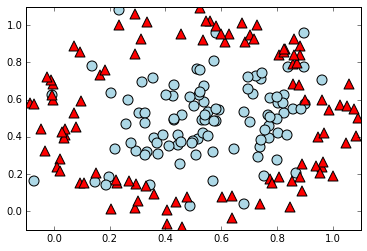The samples are not linearly separable. That is, there's no one line we can draw to cleanly separate the two classes of points.

Let's see what an SVM does on this set anyway. Below, we define a function to fit an SVM and shade part of the plane to indicate how the SVM classifies points.

In :
def fit_and_color(X,y):
# step size and limits for the meshgrid
h = 0.01
x_min, x_max = -0.1, 1.1
y_min, y_max = -0.1, 1.1
xx, yy = np.meshgrid(np.arange(x_min, x_max, h), np.arange(y_min, y_max, h))
# fit to the training data
model = LinearSVC().fit(X,y.reshape(len(X),))
# predict values for each of the mesh points
Z = model.predict(np.c_[xx.ravel(), yy.ravel()])
# color parts of the plane according to the model predictions
Z = Z.reshape(xx.shape)
plt.contourf(xx, yy, Z, alpha=0.5)

In :
fit_and_color(features,labels)
scatter(features)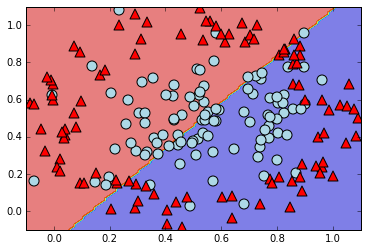As expected, the line the SVM produced doesn't seem very meaningful.

## Another dimension¶

Nevertheless, an SVM can be used to separate these points. We'll make this possible by adding one more feature: the distance to one of the sample points.

For a sense of why this is helpful, notice that adding this feature maps the samples onto a 2-d surface in 3-d space. We plot an example below, using the distance to the point (0.5, 0.5).

In :
def dist(X, p):
return (X[:,0] - p)**2 + (X[:,1] - p)**2

In :
fig = plt.figure()
X = features[:n_samples/2,:]
ax.scatter(X[:,0], X[:,1], dist(X,[0.5,0.5]), c = "lightblue", s=100, marker='o')
X = features[n_samples/2:,:]
ax.scatter(X[:,0], X[:,1], dist(X,[0.5,0.5]), c = "red", s=100, marker='^');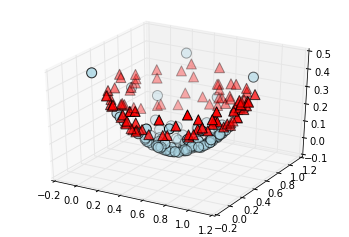The original graph of the samples corresponds to a top-down view of this plot. We can see the paraboloid surface in this 3-d plot. For another view, we can look at a projection from the side instead.

In :
X = features[:n_samples/2,:]
plt.scatter(X[:,0], dist(X,[0.5,0.5]), c = "lightblue", s=100, marker='o')
X = features[n_samples/2:,:]
plt.scatter(X[:,0], dist(X,[0.5,0.5]), c = "red", s=100, marker='^');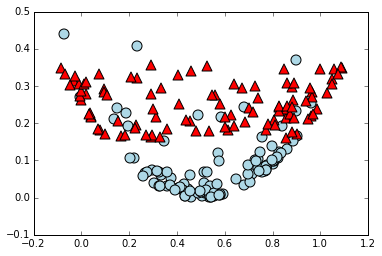From this view, it's clear that there will be an interesting linear separation to be made. We would expect an SVM to cut across the lower portion of this bowl-shape.

Below we will show the result of mapping the samples into 3-d space as above, and training the SVM on the 3-d data. This will produce a plane cutting 3-d space into two half-spaces to classify the points. To show this, we will just provide the 2-d plot given by the top-down view, and color it according to classification by the model.

The next function we define will take a matrix X whose rows are 2-d points and extend it by adding a feature to each row representing the distance of that row's point to some special points provided as an argument.

In :
def extend(X,points):
X_ext = X
for p in points:
x = p
y = p
X_ext = np.c_[X_ext, dist(X,[x,y])]
return X_ext


The next function selects n random rows from X.

In :
def random_points(X,n):
return X[np.random.randint(len(X),size=n)]


The next function extends X by computing distance to a random point in X, and then carries out our SVM fitting and plotting. The particular point that is randomly selected is essentially irrelevant. For any point, the samples will be mapped to a paraboloid with that point as the vertex, and the SVM will be able to find a plane cutting out the bulk of the blue circle points.

In :
def fit_ext_and_color(X, y, num_points = 1):
h = 0.01
x_min, x_max = -0.1, 1.1
y_min, y_max = -0.1, 1.1
xx, yy = np.meshgrid(np.arange(x_min, x_max, h), np.arange(y_min, y_max, h))
# fit model
points = random_points(X, num_points)
model = LinearSVC().fit(extend(X,points),y.reshape(len(X),))
Z = model.predict(extend(np.c_[xx.ravel(), yy.ravel()], points))
# Put the result into a color plot
Z = Z.reshape(xx.shape)
#plt.contourf(xx, yy, Z, cmap=plt.cm.Paired, alpha=0.8)
plt.contourf(xx, yy, Z, alpha=0.5)


We'll plot what the SVM comes up with for the extended feature set now.

In :
fit_ext_and_color(features,labels)
scatter(features)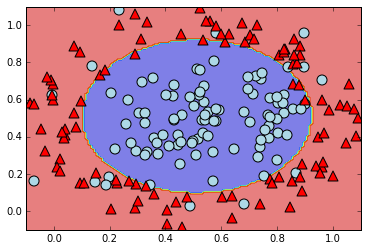The separation above corresponds to the best plane the SVM found to cut through the paraboloid we plotted in 3-d earlier.